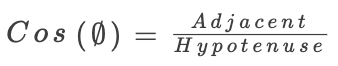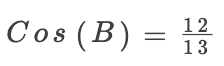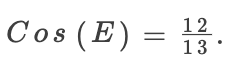### SAT数学：角度例题 3

2019-09-12 SAT-数学 阅读

# SAT Question Rank: Medium Topic: Shape, Trigonometry

Below is a question from a recent SAT exam. It is a non-calculator question that would typically take a student 1-2 minutes to complete.In the figure above, triangle ABC is similar to triangle DEF. What is the value of cos(E)?
(A)12/5
(B)12/13
(C)5/12
(D)5/13

To answer this question, we will use trigonometry. The shapes are similar which means they have the same angles. So we will work out the size of angle ABC and this will be equal to angle DEF.
For the trigonometric ratio cosine, we use the formula:The longest side, the hypotenuse, is 13. And the side Adjacent (next to) the angle is 12.
Therefore, we get the equation.And, as B and E are equal:• 地址：401-5811 Cooney Rd, Richmond, BC
• 地址：3030-8171 Ackroyd Rd, Richmond, BC
• 客服热线：604-370-0579 & 604-370-2569
• 联系邮箱：service@forbest.com

• 地址：江苏省无锡市梁溪区清扬路268号三楼
• 客服热线：181-1861-8855

•官方公众号
•加拿大官方客服
•中国官方客服